﻿ Modelling of an Explosion of an Improvised Explosive Device a Vehicle

### Modelling of an Explosion of an Improvised Explosive Device a Vehicle

Turza Jozef, Eliáš Jozef, Kopiláková Beáta, Rakúsová DankaOPEN ACCESSPEER-REVIEWED

## Modelling of an Explosion of an Improvised Explosive Device a Vehicle

Turza Jozef1,, Eliáš Jozef1, Kopiláková Beáta1, Rakúsová Danka1

1Faculty of Special Technology, Trenčín University of A. Dubček in Trenčín

### Abstract

An aim of the paper is to define behaviour of a combat vehicle based on an analysis of its movement from a point of a maximum vertical movement, acceleration and forces through development of a mathematical model of a vehicle during an operation of the vehicle in critical situations of a modern battlefield.

### At a glance: Figures

• Jozef, Turza, et al. "Modelling of an Explosion of an Improvised Explosive Device a Vehicle." American Journal of Mechanical Engineering 2.7 (2014): 209-215.
• Jozef, T. , Jozef, E. , Beáta, K. , & Danka, R. (2014). Modelling of an Explosion of an Improvised Explosive Device a Vehicle. American Journal of Mechanical Engineering, 2(7), 209-215.
• Jozef, Turza, Eliáš Jozef, Kopiláková Beáta, and Rakúsová Danka. "Modelling of an Explosion of an Improvised Explosive Device a Vehicle." American Journal of Mechanical Engineering 2, no. 7 (2014): 209-215.

 Import into BibTeX Import into EndNote Import into RefMan Import into RefWorks

### 1. Introduction

In deployment of a mechanized infantry an explosion of a charge under a vehicle may appear. The paper is aiming to make an analysis based on a developed mathematical model, what influence will it have on a vehicle’s dynamics. In the paper there is a simplified mathematical model of behaviour of the vehicle. The model can determine what acceleration (hence power) influence a vehicle and what large vertical movements may occur. An issue of a vertical movement of a vehicle after an explosion of a charge [1, 7] and in running over a bump is rather complicated; therefore some simplifications have been applied for the first analysis.

### 2. Description of Dynamics of Vehicle Movement in Explosion under a Vehicle

The BMP-2 combat vehicle has been taken for description of dynamics of a vertical movement of the vehicle, depicted with main parts needed for description of its dynamic features in the Figure 1. The traverse wheels with rockers and torque bars are important for a description, where a torque bar is a spring element for a vertical movement [1, 2, 3, 6].

An explosion of a charge under a vehicle is supposed in a lower part of a hull places under the gravity center of the vehicle.

As an issue of a movement of the vehicle is rather difficult, the following assumptions have been assumed for modelling [5, 7]:

- an explosion will occur in the middle of the vehicle under its gravity center,

- the gravity center of the vehicle is situated symmetrically in a longitudinal as well as in a transverse direction,

- we consider no longitudinal, nor transverse vibration of the vehicle, we take only vertical movement of the vehicle into consideration,

- pressure during explosion is regularly distributed on a whole lower surface of the vehicle,

- distribution of forces is regular for all loaded wheels,

- a factor of dumpers absorption is considered as different for an engaging and disengaging of a dumper,

- dumpers are constantly connected with contact points,

- terrain under vehicle is considered flat, clearance of a vehicle is the same,

- vertical movement of the vehicle downwards is limited proportionally for four rockers through lamellar and for four rockers through rubber buffers.

2.1. The Vehicle as a Whole

Mathematical description of particular important parts of the vehicle is defined in a subsequent text. Particular important elements and groups needed for description of behaviour of the vehicle are described separately with certain simplification. There are independent units, as a hull of the vehicle, rockers with torque bars, dampers, and spring and rubber buffers of the vehicle. A separate chapter is a description of a force action from an explosion of an improvised charge on a vehicle bottom.

2.2. Vehicle Hull

With respect to the assumptions we are taking into consideration the forces are acting symmetrically only in a cross and longitudinal sections. The action of forces acting on the vehicle hull in a cross and longitudinal sections are illustrated in the Figure 2. There are forces from placing a torque bar FA, from the weight of the vehicle Gv, a force from a vehicle acceleration Fmv, forces from dampers Ftl from the first and the sixth pair of wheels, forces from a spring buffer Fpr1 in the first and the sixth pair of wheels, forces from a spring buffer Fpr2 in the second and the fifth pair of wheels having the number of rockers with wheels nk=12.

From a balance of forces in a vertical direction the following relation applies.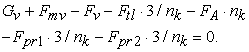(1)

Gravitational force of the vehicle having an mv weight is defined as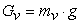(2)

and an accelerating force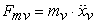(3)

The other forces and relations will be defined in the following text.

2.3. A Rocker with a Traverse Wheel and a Torque Bar

We suppose that a road has a flat surface. Kinematics of a movement and main parameters of a traverse wheel rocker with a torque bar is illustrated in Figure 3. We can simplify it for purposes of a dynamic analysis with a certain approximation as in Figure 4. An action of forces on a rocker in a vertical plane is illustrated in Figure 5. Let us simplify and choose a simulated rocker that the forces of a dumper, the forces of a spring and rubber buffer having a size with 4/nk ratio, are acting.

Then in a vertical plane there are acting forces on a simulated rocker as by Figure 5. Particularly there are defined the Mt momentum of a torque bar and a MI momentum from a rotation of a rocker. An Fc force from a road at a1 distance implies an additional bending moment on a rocker and therefore a deformation as well. With regard to a massiveness of a rocker we are not taking its deformation into consideration for our purposes. We do not consider any vertical roughness of a road. The description is made for a group of a simulated rocker of a traverse Wheel with a torque bar. Through an implementation of a course of a vertical movement due to a road roughness, a dynamic behaviour of a driving vehicle could be determined.

In Figure 5 there are illustrated all stresses applied to the rocker in a vertical plane. The principle of a movement of a rocker, damper, a rubber and a spring buffer is delineated there. The movement will be explored only on the only one rocker. The number of rockers is n=12, number of spring buffers is four and number of rubber buffers is four. The weight of a vehicle per a rocker is reduced in 1/nk ratio, therefore the number of buffers is also considered in 1:4 ratio. An uplift of a rocker (vehicle) will be considered approximately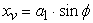(4)

The Mt torque of a torque bar, having an lt length of a torque bar, the Gt modulus of elasticity in shear and a Jt polar square moment of a torque bar cross-section having a dt diameter causes twisting of a rocker by an angle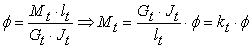(5)

where a polar square moment of a torque bar cross-section is defined from a relation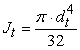(6)

Stiffness of a rocker torque bar is defined using an equation (5)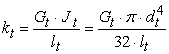(7)

There are applied similar relations between a twisting φ and a shift of a vehicle body in large and small deformations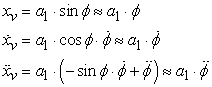(8)

We suppose balanced loading of all rockers of traverse wheels with torque bars. A reduced Ir moment of inertia for a rotational movement of a rocker with a Wheel is calculated based on a relation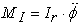(9)

where Ir is a mass moment of inertia of a rocker arm o a traverse wheel and a torque bar. For a vertical movement of an A point (Figure 5) the equation of a balance in a vertical direction is applied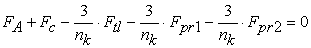(10)

a torque equation to A point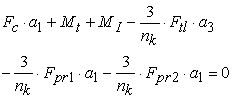(11)

respectively to B point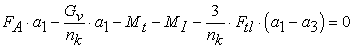(12)

The buffer forces Fpr1 and Fpr2 start acting only after having finished an xmax uplift (Figure 3). A mass moment of inertia for Ivr rocker is computed based on a relation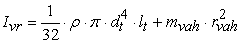(13)

After having substituted real values for simulation we receive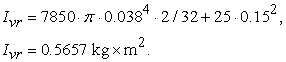(14)
2.4. Damper

It is a double action hydraulic damper, the manufacturer states from testing with 40 double lifts per minute its mean power 3.25 kN in piston rod disengagement and 13 kN in piston rod engagement at ht=0.1 m uplift. As a speed of a piston rod uplift in testing is the same in engagement and disengagement, time of uplift is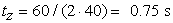(15)

and a speed of uplift is(16)

a coefficient of a damping the piston rod down in disengaging is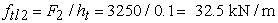(17)

and a coefficient of a damping the piston rod down in engaging is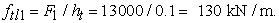(18)

A force of a damper can be expressed by an equation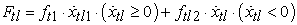(19)

An uplift of a damper on a rocker will be approximately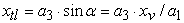(20)
2.5. Spring Buffer

A plate spring has been used in form of an Archimedean spiral in conformity with Figure 7. Basic dimensions for computation were: number of convolutions nl=7, final angle of a spiral φp1=0.1 rad, φp2=0.1833 round, plate width hl=92 mm, plate thickness bl=4 mm, maximum compression xdm=86.6 mm, radius of a spiral origin r1=12 mm, radius of a spiral end r2=44 mm, modulus of elasticity of a plate material El=210 GPa.

A square moment of a cross-section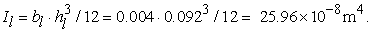(21)

Length of a spiral plate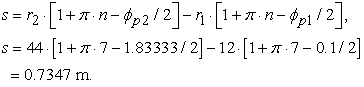(22)

Length of a plate of an Archimedean spiral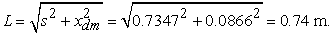(23)

Stiffness of a plate spring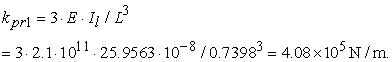(24)

After having finished an uplift of a spring, stiffness of a buffer will be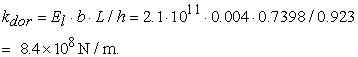(25)

Course of a spring force will be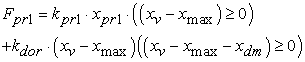(26)

in compression of a plate spring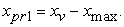(27)

Course of forces of a plate spiral spring and a fixed buffer is illustrated in the Figure 8.

2.6. Rubber Buffer

Construction of a rubber buffer is illustrated in the Figure 9. A rubber is vulcanically connected on a segment. A segment is firmly attached to a vehicle hull with a bolted connection. The dimensions and data on a rubber in accordance with the manufacturer’s data are ag1=60 mm, ag2=70 mm, bg1=50 mm, bg2=60 mm, hg=53 mm. A dynamic modulus of elasticity in pressure for a used rubber is considered to be Eg=10 MPa.

Compression of a rubber buffer is computed through a relation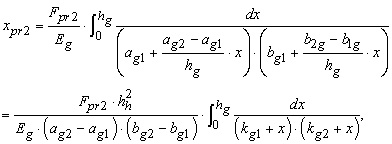(28)

Where k1, k2 are auxiliary constants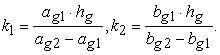(29)

Through an integration of an equation 28 and using constants from an equation 29 we receive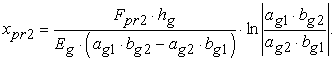(30)

From equation 30 after an adjustment a stiffness of a rubber buffer can be obtained in a following form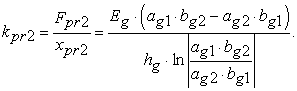(31)

After having substituted the dimensions and parameters of a rubber buffer into an equation 31 we receive an intensity of stiffness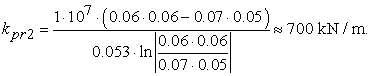(32)

Relation for computation of a force of a rubber buffer will be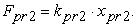(33)

Course of a force of a rubber buffer is drawn by an equation 33 in the Figure 10.

2.7. Forces from an Explosion on a Vehicle

An overpressure spreads on a front of a blast wave in an explosion of a charge in spherical surfaces. The time of a rise and a discharge of a blast wave are very short, in order up to 20 ms. Makovička was dealing with modelling of a course of a blast wave in more details  and he defined the most probable course. His model has been used in the next text for our case. A value of an overpressure on a front of a blast wave will be calculated through following relations. A reduced spacing distance from an Rp explosion epicenter will be calculated through a distance from an epicenter of an R charge explosion in m, a total equivalent mass of a charge Cw in m/kg1/3 from a relation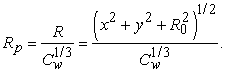(34)

There is a different value of an overpressure (Figure 11) acting a each point P on a bottom of a vehicle having dimensions av, bv on an element of a surface dA=dxdy at R distance. This value will be calculated from a relation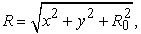(35)

where R0 is a vertical distance of a lower surface of the vehicle from a road, x, y are coordinates of the P point in a coordinate system x, y.

A total equivalent mass of a charge in kg TNT (trinitrotoluene) is defined from a relation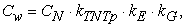(36)

Where CN is a weight of a used charge of an explosive in kg, kNTp is a pressure TNT equivalent, kE coefficient of a charge sealing, kG coefficient of a geometry of a blast wave spreading in a space (for a detonation in a free space kG=1, on a terrain surface kG=2), kB is a ballistic ratio (ratio of a case weight in kg/ to weight of explosives in kg). A sealing coefficient is defined based on relation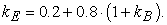(37)

A size of an overpressure in MPa on a front of a blast wave for a ground explosion in MPa at a ground explosion in external environment for Rp≤1 (in our case the term is applied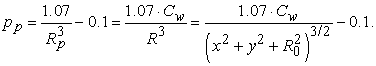(38)

An overpressure in an overpressure phase of a blast wave in Mpa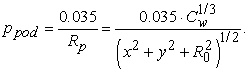(39)

Duration of an overpressure wave in s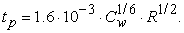(40)

Duration of an under pressure wave in s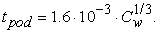(41)

Course of an overpressure and an under pressure wave in time by previous relations is illustrated in figure, where p0 is a pressure of an environment. For different 4 values of a charge weight in kg of TNT a Figure 13 illustrates a maximum value of an overpressure for a vertical distance from a bottom surface of vehicle from a road R0=0.5 m.

In a selected I quadrant a bottom of a vehicle is divided through a grid into ten sections in a cross direction of a vehicle (x=1÷10) and also into ten sections in a longitudinal direction (y=1÷10).

Figure 14 illustrates a course of maximum overpressures in a cross-section in tp time and the Figure 15 in a longitudinal direction.

Figure 16. Spatial representation of a maximum value of a pressure in the I quadrant in a cross and longitudinal directions

Based on courses we can note, that if a blast wave leaves in a longitudinal direction, the value of a maximum pressure is lower than if it leaves under a vehicle in a cross direction. It relates a symmetric action of an overpressure on a bottom part of the vehicle, therefore a calculation can be done only for one quadrant, and for example the first one and a resulting force will be multiplied by 4 x. A value of an under pressure is very small, therefore it will be neglected for a next calculation of forces from a charge explosion. A size of maximum acting force acting on a bottom of a vehicle in tp time in accordance with Figure 11 can be defined based on a relation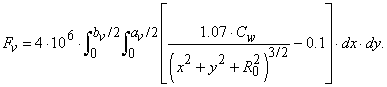(42)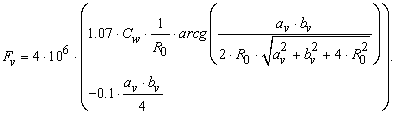(43)

In case, we would considered, that under a whole surface of a vehicle there is acting a maximum overpressure, a value of a maximum force in tp time would be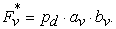(44)

A course of a force is acting on a bottom of a vehicle in tp time only with a maximum value of Fv in time tp/2, it means in a form of an isoscelent pulse (Figure 12, respectively for particular values by a Figure 13). With respect to small dimensions of a vehicle we do not consider any regressive blast wave.

For combat vehicles we need to consider mostly the mass of a used charge up to CN=5 kg TNT placed just about a terrain surface, where kG=2.

### 3. Simulation Model

A simulation model has been made up in a computation model DYNAST based on above mentioned equations for a particular vehicle through impedance networks. A block simulation model is shown in the Figure 17. Stiffness of a torque bar with a rocker using equations 5 and 7 with a length of a torque bar lt=1.93 m, its modulus of elasticity in a material shear Gt=78.5 GPa and a diameter dt=38 mm is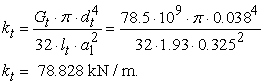(45)

In balanced stable conditions a φst deformation of rockers is defined using equations 8 and 11, equations 11 and 12 and further adjustment without taking forces of a damper and buffers into consideration as follows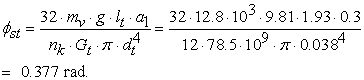(46)

with a corresponding angle φst=2.202°, respectively a static compression in accordance with equation 8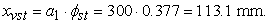(47)

This value will be an initial condition for a further solution in a simulation. The previous values were calculated for a length of a torque bar lt=1.93 m, diameter of a torque bar dt=38 mm, weight of a vehicle mv=12800 kg, gravity acceleration g=9.81 m/s2.

Aiming to form a block simulation modul there were used an Rtl movement resistance observing a damping feature of a damper, an Lt acceleration resistance describing a mass moment of inertia of a rocket and attached masses, Ct capability of a torque bar observing stiffness of a torque bar, a Epr1 source respecting a feature of a spring buffer, a Epr2 observes a feature of a rubber buffer, Lv an acceleration resistance observing a mass of a vehicle, the Epm source observes a mass of a vehicle, Ev source of force from explosion, J1 and E1 represent a transformer of a vehicle movement and a rocker movement, BIv and BIt are integrating blocks to define uplifts of a vehicle xv and uplift at the end of a rocker xt. Theory in more details is mentioned in .

### 4. Results of a Simulation

Some results of a simulation of the explosion under a vehicle with a charge of size CN=5 kg TNT in accordance with data from a previous text are drawn in the Figure 18.

Figure 18. Results of simulation of a charge explosion under a vehicle

A course xv of a vehicle uplift is drawn in the Figure18, having a vv speed of the uplift and an av acceleration in a vertical direction during an explosion of a charge under a centre of a vehicle. From courses it can be seen that a maximum uplift of a vehicle is 388 mm at 10.8 ms, a minimum uplift -115 mm, becoming stabilized after 1.5 s. Speed of uplift is maximum at 5.873 m/s in time 16.5 ms and minimum -1.419 m in time 1.5 s. A maximum value of acceleration is 6542 m/s2 in time of 1 ms and minimum -46.92 m/s2 in time of 56.5 ms. A value of a maximum acceleration for a charge being considered is significant and it has a great influence on a vehicle crew. The values would be lesser if also deformation and damping features of the vehicle hull would be taken into consideration. At computed accelerations it would be difficult to design the seats of a crew that would eliminate an effect of acceleration.

Size of a maximum force at explosion would be Fvm= 7.69 MN of a saw-tooth pulse lasting tmax= 1.66 ms.

From values of courses in the Figure 18 we can note that kinematics, used stiffness of rockers and dampers of the vehicle are designed very well and they provide for a strong aperiodic course without additional vibration after a vehicle incidence.

### 5. Conclusions

A generated simplified mathematical model and results of a simulation have shown that a construction of a chassis is very well designed.

It has been proved that action by force from an explosion of a charge of a size of CN= 5 kg TNT is significant, mainly a value of an acceleration of a vertical movement of a vehicle.

Based on verification by a created program we are presenting its improvements also for an explosion of a charge aside from a vehicle centre.

### Acknowledgement

This publication was created in the frame of the project "Alexander Dubček University of Trenčín wants to offer high-quality and modern education", ITMS code 26110230099, based on the Operational Programme Education and funded from the European Social Fund.

### References

  Eliaš, J. 2013: Zvýšenie bezpečnosti členov roja bojového vozidla pri jazde v ťažkých terénnych podmienkach moderného bojiska. (Increase the safety of members combat vehicle group when driving in off road conditions of the modern battlefield). In: Zborník prednášok zo 14. medzinárodnej vedeckej konferencie Transfér 2013, 17-18. október 2013. Trenčín, FŠT TnU AD 2013.In article  Eliaš, J., 2002. Špeciálna mobilná technika na pásových podvozkoch: Charakteristiky, Technické údaje a popis. (Special mobile equipment tracked: Features, Specifications and description.) Issue 1. Trenčín: TnU, 2002.In article  Ferencey, V., Dropa, P. 2005. Mechanika pohybu pásovej mobilnej techniky. (Mechanics of movement band mobile technology). Trenčín: TnU, 2005.In article  Makovička, D, Makovička, D. ml.: Design of bulding structure loaded by explosion shock wave. Available. http://www.makovicka.cz/publikace/2009_po_cz.pdf.In article  Skřehot, P. 2009. Prevence nehod a havárií. Časopis výzkumu a aplikací v profesionální bezpečnosti. (Prevention of incidents and accidents. Magazine research and applications in the professional security). 2009, roč. 2, č. 4.In article  Sloboda, A., Ferencey, V., Hlavňa, V., Tkáč, Z.. 2008: Konštrukcia kolesových a pásových vozidiel. (The design of wheeled and tracked vehicles). Vienala, Košice 2008.In article  Turza, J., Eliaš, J. 2013: (Analysis of dymamic features of mine trap effects on a crew of a combat vehicle). In: Zborník prednášok zo 14. medzinárodnej vedeckej konferencie Transfér 2013, 17-18. október 2013. Trenčín, FŠT TnU AD 2013.In article  Turza, J., Tkáč, Z., Vanc, M., Bucha, J., Rybičková, L., Čelko, P., Kleinedler, P. 2009. Axiálne piestové hydrostatické prevodovníky: Teória a výpočet. (Axial piston hydrostatic pump and motor. Theory and calculation.). Issue 1 - Trenčín: Trenčianska univerzita Alexandra Dubčeka, 2009.In article